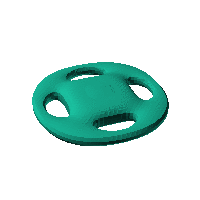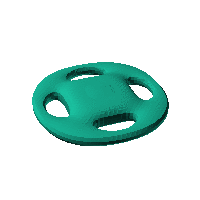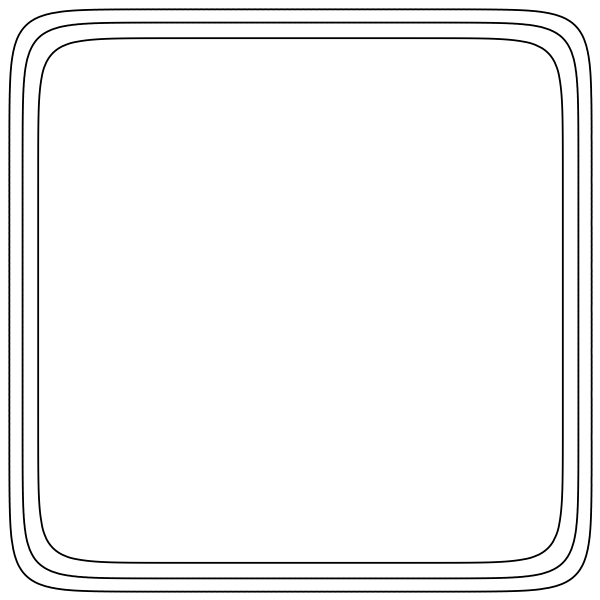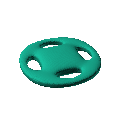Blob Farm# blob23The blob in all its glory:circular case with 4 handles.

 Click on the snapshot to download the blob's stl file.Octave Code:
```1; # Prevent Octave from thinking that this is a function
# though one is defined here

function w = f(x2,y2,z2,c,r,e)
x  = (x2-c(1))/r(1);
y  = (y2-c(2))/r(2);
z  = (z2-c(3))/r(3);
# function at origin must be <0, and >0 far enough away.  w=0 defines the surface
k2 = 9.3;

nb = 4;
th = atan2(x,y);
r = sqrt(x.^2+y.^2);
w = 3./((1+0.5*cos(nb*th)).^2 + (z/2).^2 + (r-5).^2) - 1./((x/k2).^2+(y/k2).^2+(z/1.5).^4)+1 ;

endfunction;

```
GNU Octave